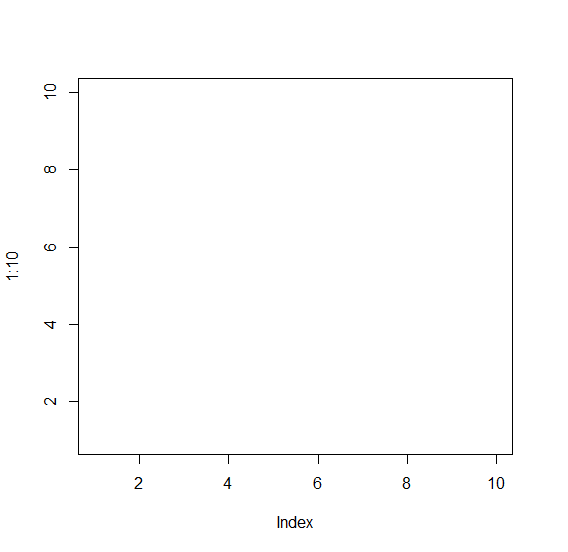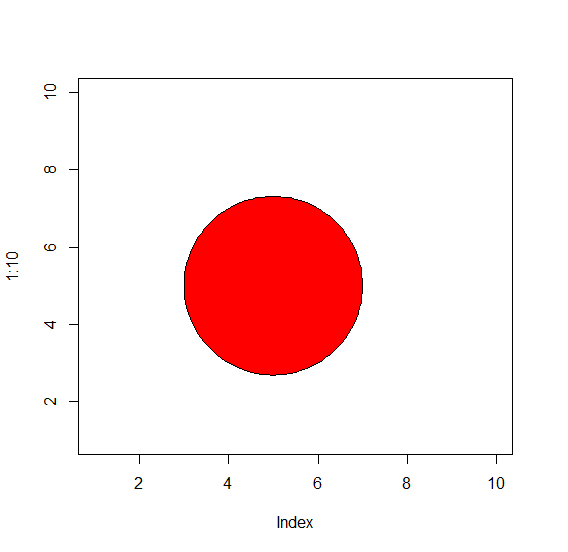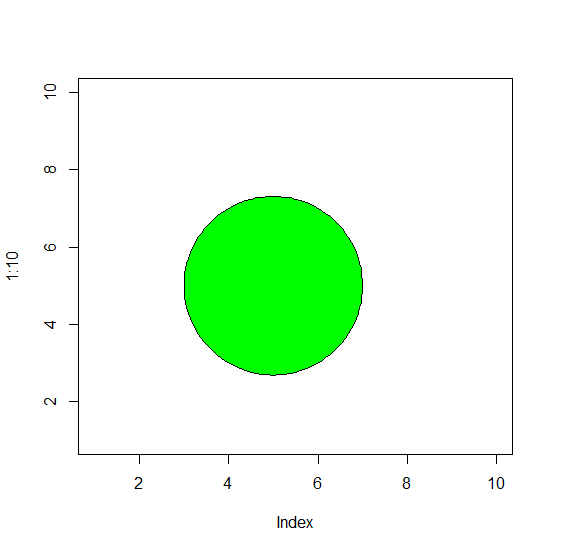# How to create a circle filled with a color in R?

We can create a circle in R by using draw.circle function of plotrix package and default color of the circle is white. If we want to change the color of a circle then we can use col argument and pass the desired colors.

For Example, if we want to create a blue colored circle then we can use the command given below −

draw.circle(5,5,2,col="blue")

Check out the below Example to understand how it works.

## Example

To create a circle filled with a colour, use the command given below −

plot(1:10,type="n")


## Output

If you execute the above command, it generates the following Output −To create a circle filled with a colour, add the following code to the above command −

plot(1:10,type="n")
library(plotrix)
draw.circle(5,5,2,col="red")

## Output

If you execute all the above given commands as a single program, it generates the following Output −To create a circle filled with a colour, add the following code to the above command −

plot(1:10,type="n")
library(plotrix)
draw.circle(5,5,2,col="green")


## Output

If you execute all the above given commands as a single program, it generates the following Output −